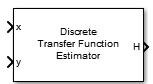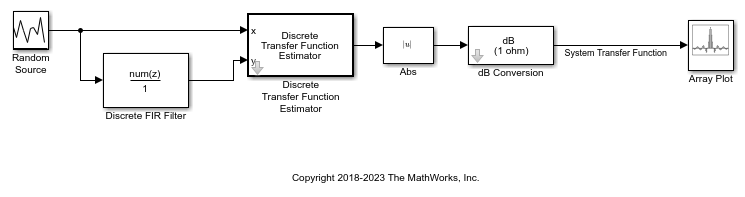# Discrete Transfer Function Estimator

Compute estimate of frequency-domain transfer function of system

•Libraries:
DSP System Toolbox / Estimation / Power Spectrum Estimation

## Description

The Discrete Transfer Function Estimator block estimates the frequency-domain transfer function of a system using the Welch’s method of averaged modified periodograms.

The block buffers the input data into overlapping segments. You can set the length of the data segment and the amount of data overlap through the parameters set in the block dialog box. The sample rate of the block is equal to 1/T. T is the sample time of the inputs to the block.

The block first applies a window function to the two inputs, x and y, and then scales them by the window power. It takes the FFT of each signal, calling them X and Y. The block calculates Pxx which is the square magnitude of the FFT, X. The block then calculates Pyx which is X multiplied by the conjugate of Y. The output transfer function estimate, H, is calculated by dividing Pyx by Pxx.

## Examples

expand all

This example shows how to use the Discrete Transfer Function Estimator block to estimate the frequency-domain transfer function of a system.

Open the model. The Random Source block represents the system input signal. The sample rate of the system input is 44.1 KHz. The Random Source input passes through a low-pass filter with a normalized cutoff frequency of 0.3. The filtered signal represents the system output signal. Because the Discrete Transfer Function Estimator block outputs complex values, take the magnitude of the output to see a plot of the transfer function estimate.

Run the model. The transfer function plot displays the system transfer function, a low-pass filter that matches the frequency response of the Discrete FIR Filter block.## Ports

### Input

expand all

Specify the system input signal as a vector or a matrix.

The inputs x and y must have the same size and data type.

Data Types: `double` | `single`

Specify the system output signal as a vector or a matrix.

The inputs x and y must have the same size and data type.

Data Types: `double` | `single`

### Output

expand all

Transfer function estimate, returned as a vector or a matrix. For more details on how the block computes the transfer function estimate, see Algorithms.

Data Types: `double` | `single`

## Parameters

expand all

Specify the source of the window length value. You can set this parameter to:

• `Same as input frame length` — Window length is set to the frame size of the input.

• `Specify on dialog` — Window length is the value you specify in Window length.

Specify the length of the window, in samples, used to compute the spectrum estimate as a positive integer greater than or equal to `2`.

#### Dependencies

To enable this parameter, set Window length source to ```Specify on dialog```.

Specify the percentage of overlap between successive data windows as a nonnegative scalar in the range [`0`,`100`).

Specify the averaging method as `Running` or `Exponential`. In the running averaging method, the block computes an equally weighted average of specified number of spectrum estimates defined by Number of spectral averages parameter. In the exponential method, the block computes the average over samples weighted by an exponentially decaying forgetting factor.

Select this check box to specify the forgetting factor from an input port. When you do not select this check box, the forgetting factor is specified through the Forgetting factor parameter.

#### Dependencies

To enable this parameter, set Averaging method to `Exponential`.

Specify the exponential weighting forgetting factor as a positive scalar ≤ `1`.

#### Dependencies

To enable this parameter, set Averaging method to `Exponential` and clear the Specify forgetting factor from input port parameter.

Specify the number of spectral averages as a positive integer. The Transfer Function Estimator block computes the current estimate by averaging the last N estimates. N is the number of spectral averages.

#### Dependencies

To enable this parameter, set Averaging method to `Running`.

Specify the source of the FFT length value as `Auto` or `Property`.

When the source of the FFT length is set to `Auto`, the block sets the FFT length to the input frame size. When the source of the FFT length is set to `Property`, you specify the FFT length in the FFT length parameter.

Specify the length of the FFT that the Discrete Transfer Function Estimator block uses to compute spectral estimates as a positive integer.

Specify a window function for the Discrete Transfer Function Estimator block. Possible values are:

• `Hann`

• `Chebyshev`

• `Flat Top`

• `Hamming`

• `Kaiser`

• `Rectangular`

Specify the sidelobe attenuation of the window as a positive scalar in dB.

#### Dependencies

To enable this parameter, set Window function to `Kaiser` or `Chebyshev`.

Specify the frequency range of the transfer function estimate.

• `Centered` –– The block computes the centered two-sided transfer function of the real or complex input signals, x and y.

• `onesided` –– The block computes the one-sided transfer function of real input signals, x and y.

• `twosided` –– The block computes the two-sided transfer function of the real or complex input signals, x and y.

Select this check box to compute and output the magnitude squared coherence estimate using Welch’s averaged, modified periodogram method. The magnitude squared coherence estimate indicates how well two inputs correspond to each other at each frequency.

Type of simulation to run. You can set this parameter to:

• `Code generation` –– Simulate model using generated C code. The first time you run a simulation, Simulink® generates C code for the block. The C code is reused for subsequent simulations, as long as the model does not change. This option requires additional startup time but provides faster simulation speed than ```Interpreted execution```.

• `Interpreted execution` –– Simulate model using the MATLAB®  interpreter. This option shortens startup time but has slower simulation speed than ```Code generation```.

## Block Characteristics

 Data Types `double` | `single` Multidimensional Signals `No` Variable-Size Signals `No`

expand all

## Version History

Introduced in R2014a